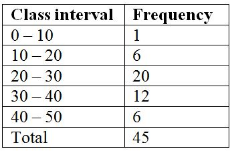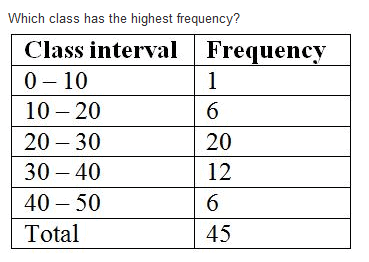Courses

# Test: Data Handling- 1

## 10 Questions MCQ Test Mathematics (Maths) Class 8 | Test: Data Handling- 1

Description
This mock test of Test: Data Handling- 1 for Class 8 helps you for every Class 8 entrance exam. This contains 10 Multiple Choice Questions for Class 8 Test: Data Handling- 1 (mcq) to study with solutions a complete question bank. The solved questions answers in this Test: Data Handling- 1 quiz give you a good mix of easy questions and tough questions. Class 8 students definitely take this Test: Data Handling- 1 exercise for a better result in the exam. You can find other Test: Data Handling- 1 extra questions, long questions & short questions for Class 8 on EduRev as well by searching above.
QUESTION: 1

### The number of times an observation occurs in a data is called its

Solution:

The frequency  of a particular data value is the number of times the data value occurs. For example, if four students have a score of 80 in mathematics, and then the score of 80 is said to have a frequency of 4. The  frequency of a data value is often represented by ' f '.

QUESTION: 2

### In the class- interval 70-80, 80 is the

Solution:

Upper limit is the highest number of the interval. Hence 80 is the upper limit.

QUESTION: 3

### The class mark of 95-100 is

Solution:

Class mark is the midpoint of the upper and lower limits.
so for the given problem;

the class mark is
[ (95+100)÷2 ] = 97.5
Thus class mark is 97.5

QUESTION: 4

The difference between the upper and lower limit is called

Solution:

Class Size: Difference between the true upper limit and true lower limit of a class interval is called the class size.

Class size remains the same for all class intervals.

For the class interval 10 - 20

Class size is 10, i.e., (20 - 10 = 10)

QUESTION: 5

The shape of a pie-chart is

Solution:

A pie chart is circular in shape which uses pie slices to show relative sizes of data

QUESTION: 6

The pie-chart is divided into

Solution:

A pie chart is a circular chart divided into wedge-like sectors, illustrating proportion. Each wedge represents a proportionate part of the whole, and the total value of the pie is always 100 percent. Pie charts can make the size of portions easy to understand at a glance.

QUESTION: 7

The central total angle in a pie chart is

Solution:
QUESTION: 8

18 out of 36 people love reading, so reading in the pie chart will be represented by

Solution:

When we find the central angel on pie chart we get 18/36*360•=180•which is half of pie.so we write that semi circular sector love reading.

QUESTION: 9

What is the size of class intervals?Solution:
QUESTION: 10Solution:

20 has the highest frequency as option b because as you have seen and frequency table the highest frequency is friendly only so it shows that it has the highest frequency and don't you feel bad I may tell you one thing this thing should not be asked you know these are very easy and you can see just a frequency table.